Latest Banking jobs   »   Twisted One Reasoning Ability Quiz for...

# Twisted One Reasoning Ability Quiz for All Banking Exams- 14th May

Directions (1-5): There are two rows given and to find out the resultant of a particular row we need to follow the following steps: –
Step 1: If an even number is followed by an odd number then the resultant will be the addition of both the numbers.
Step 2: If an odd number is followed by a perfect square then the resultant will be the difference of that square number from the odd number.
Step 3: If an odd number is followed by another odd number (but not a perfect square) then the resultant will be the addition of both the numbers.
Step 4: If an odd number is followed by an even number (but not a perfect square) then the resultant comes by multiplying the numbers.
Step 5: If an even number is followed by another even number then the resultant will be the division of first number by the second number.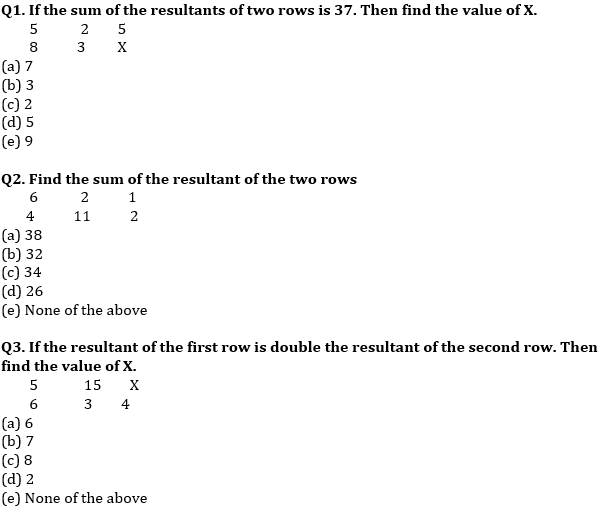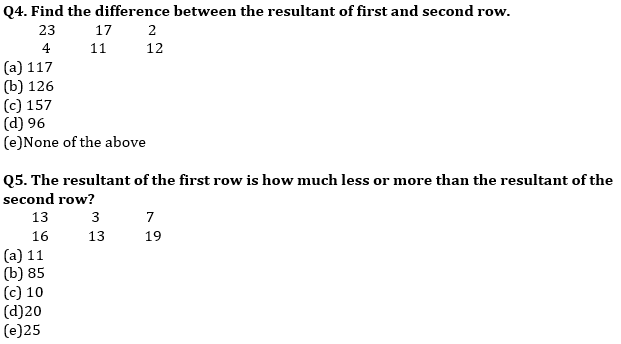Directions (6-10): In each of the questions below are given some statements followed by some Conclusions. You have to take the given statements to be true even, if they seem to be at variance from commonly known facts. Read all the conclusions and then decide which of the given conclusions logically follows from the given statements disregarding commonly known facts.
(a) If only conclusion I follows.
(b) If only conclusion II follows.
(c) If either conclusion I or II follows.
(d) If neither conclusion I nor II follows.
(e) If both conclusions I and II follow.

Q6. Statements:
Some Pen are Green
Only green are Potato
Only a few Green are mango
Conclusions:
I: All green can be mango
II: Some potato is pen is a possibility

Q7. Statements:
All North are south
Only East is west
No East is north
Conclusions:
I: Some South is west
II: Some south can be east

Q8. Statements:
Only a few Hindi are Physics
Some Physics are Science
All Physics is Mathematics
Conclusions:
I: All mathematics is Hindi is a possibility
II: Some Science is Mathematics

Q9. Statements:
Some Apple are Orange
All Pink is Orange
No Orange is Brown
Conclusions:
I: No pink is brown
II: Some pink are apple

Q10. Statements:
All Red is blue
No blue is Green
Only a few green is white
Conclusions:
I: All Red can never be Green
II: Some white can be blue

Directions (11-15): In each question below are given some statements followed by some conclusions. You have to take the given statements to be true even if they seem to be at variance with commonly known facts. Read all the conclusions and then decide which of the given conclusions logically follows from the given statements, disregarding commonly known facts.

Q11. Statements:
Only a few paper are colour.
No picture is colour.
All paper is nature.
Conclusions:
I. All picture are nature is a possibility.
II. No nature is colour.
(a) Only I follows
(b) Both I and II follow
(c) Only II follows
(d) None follows
(e) None of these

Q12. Statements:
No winter is coffee.
Some coffee are hot.
All tea are winter
Conclusions:
I. All hot is winter is a possibility
II. Some tea is coffee
(a) Only I follows
(b) Both I and II follow
(c) Only II follows
(d) Either I or II follows
(e) None follows

Q13. Statements:
Only purple are Orange.
No Purple is Green.
Only a few Green are White.
Conclusions:
I. Some Green is Orange
II. Some White is purple is a possibility
(a) Only II follows
(b) Both I and II follow
(c) Only I follows
(d) Either I or II follows
(e) None follows

Q14. Statements:
All Stone are river.
Some marble are mountain.
All river are marble.
Conclusions:
I. Some mountain are Stone.
II. All mountain are river is a possibility.
(a) Only I follows
(b) None follows
(c) Both I and II follow
(d) Only II follows
(e) None of these

Q15. Statements:
Some fruit are flower.
All tree are flower.
No plant is tree.
Conclusions:
I. All plant is flower is a possibility
II. All plant is fruit is a possibility
(a) Only II follows
(b) Both I and II follow
(c) None follows
(d) Either I or II follows
(e) None of these

Practice More Questions of Reasoning for Competitive Exams:

Solutions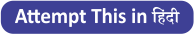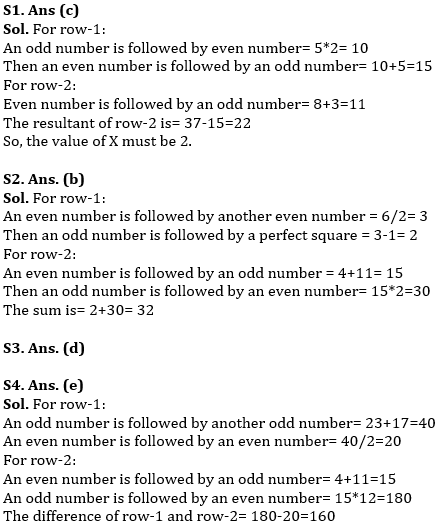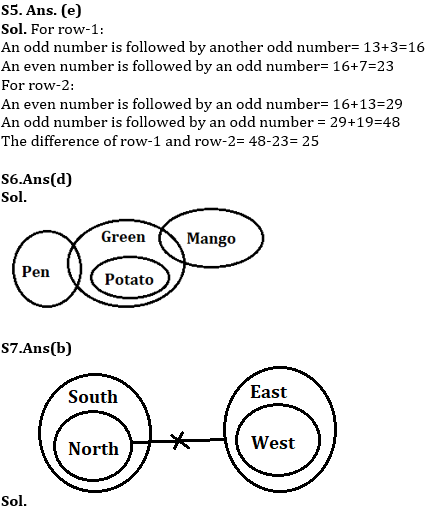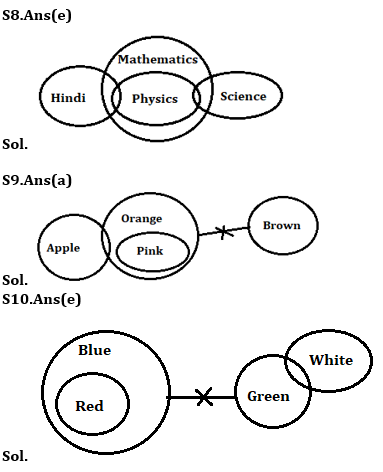#### Congratulations!